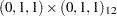The ARIMA Procedure

## Example 7.7 Iterative Outlier Detection

This example illustrates the iterative nature of the outlier detection process. This is done by using a simple test example where an additive outlier at observation number 50 and a level shift at observation number 100 are artificially introduced in the international airline passenger data used in Example 7.2. The following DATA step shows the modifications introduced in the data set:

```data airline;
set sashelp.air;
logair = log(air);
if _n_ = 50 then logair = logair - 0.25;
if _n_ >= 100 then logair = logair + 0.5;
run;
```

In Example 7.2 the airline model, ARIMA, was seen to be a good fit to the unmodified log-transformed airline passenger series. The preliminary identification steps (not shown) again suggest the airline model as a suitable initial model for the modified data. The following statements specify the airline model and request an outlier search.

```/*-- Outlier Detection --*/
proc arima data=airline;
identify var=logair( 1, 12 )  noprint;
estimate q= (1)(12) noint method= ml;
outlier maxnum=3 alpha=0.01;
run;
```

The outlier detection output is shown in Output 7.7.1.

Output 7.7.1 Initial Model
 SERIES A: Chemical Process Concentration Readings

The ARIMA Procedure

Outlier Detection Summary
Maximum number searched 3
Number found 3
Significance used 0.01

Outlier Details
Obs Type Estimate Chi-Square Approx Prob>ChiSq
100 Shift 0.49325 199.36 <.0001
50 Additive -0.27508 104.78 <.0001
135 Additive -0.10488 13.08 0.0003

Clearly the level shift at observation number 100 and the additive outlier at observation number 50 are the dominant outliers. Moreover, the corresponding regression coefficients seem to correctly estimate the size and sign of the change. You can augment the airline data with these two regressors, as follows:

```data airline;
set airline;
if _n_ = 50 then AO = 1;
else AO = 0.0;
if _n_ >= 100 then LS  = 1;
else LS = 0.0;
run;
```

You can now refine the previous model by including these regressors, as follows. Note that the differencing order of the dependent series is matched to the differencing orders of the outlier regressors to get the correct "effective" outlier signatures.

```/*-- Airline Model with Outliers --*/
proc arima data=airline;
identify var=logair(1, 12)
crosscorr=( AO(1, 12) LS(1, 12) )
noprint;
estimate q= (1)(12) noint
input=( AO LS )
method=ml plot;
outlier maxnum=3 alpha=0.01;
run;
```

The outlier detection results are shown in Output 7.7.2.

Output 7.7.2 Airline Model with Outliers
 SERIES A: Chemical Process Concentration Readings

The ARIMA Procedure

Outlier Detection Summary
Maximum number searched 3
Number found 3
Significance used 0.01

Outlier Details
Obs Type Estimate Chi-Square Approx Prob>ChiSq
135 Additive -0.10310 12.63 0.0004
62 Additive -0.08872 12.33 0.0004
29 Additive 0.08686 11.66 0.0006

The output shows that a few outliers still remain to be accounted for and that the model could be refined further.Previous Page | Next Page | Top of Page